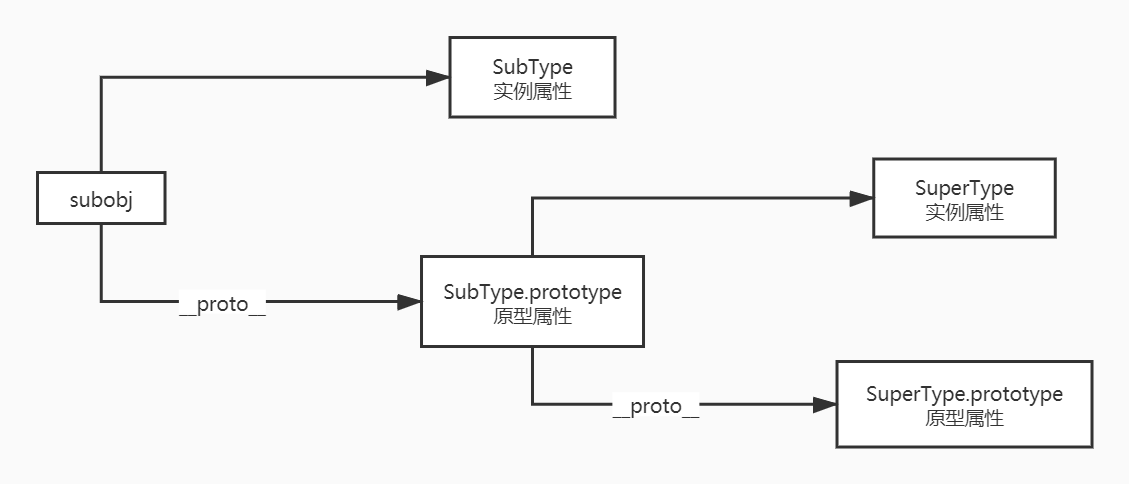# JS 继承方法详解

## new 操作的实现原理

• `obj` 具有 `SuperType` 所有的实例属性、方法和原型属性、方法
• `obj.constructor === SuperType`, 返回 true
• `obj.__proto__ == SuperType.prototype`, 返回 true
• `obj` 上的原型方法与 `SuperType` 原型上的同一方法在内存上应该一致
• `new` 操作最后返回了一个对象
• `obj instanceof SuperType`, 返回 true

``````function SuperType() {
this.prop = true;
this.func = function () {};
}

SuperType.prototype.protofunc = function () {};
``````

``````var obj1 = new SuperType();

console.log(obj1.constructor === SuperType); // true
console.log(obj1.__proto__ == SuperType.prototype); // true
console.log(obj1.protofunc === SuperType.prototype.protofunc); // true
console.log(obj1 instanceof SuperType); // true
``````

``````function newinstance(Type) {
// 首先创建一个对象实例，该对象包括一个 __proto__ 属性，需要指向 Type.prototype
var o = {
__proto__: Type.prototype,
};

// 接着，对象实例 o 需要具有 Type 的所有实例属性和原型属性
// 即，在o对象实例上运行 Type 的构造函数，初始化 Type 的那些属性和方法
Type.apply(o);

// 最后，需要返回这个对象实例
return o;
}
``````

``````// 首先定义函数printAllProps()，用于输出所有可枚举的实例属性、原型属性
// 不用console.log输出的原因见获得对象属性的方法的博文
function printAllProps(obj) {
let array = [];
for (let prop in obj) {
array.push(prop);
// 如果要输出属性值，使用：
// array.push(prop + ':' + obj[prop])
}
console.log(array);
}
``````
``````var obj = newinstance(SuperType);
printAllProps(obj);
``````

``````console.log(obj.constructor === SuperType); // true
console.log(obj.__proto__ == SuperType.prototype); // true
console.log(obj.protofunc === SuperType.prototype.protofunc); // true
console.log(obj instanceof SuperType); // true
``````

## 使用原型链进行继承

``````function SuperType() {
this.prop = true;
this.func = function () {};
}

SuperType.prototype.protofunc = function () {};

function SubType() {
this.subprop = true;
this.subfunc = function () {};
}

SubType.prototype = new SuperType(); // !important

var subobj = new SubType();
````````````SubType.prototype = new SuperType();

// 错误方法
// SubType.prototype = {
//     newfunc: function () {},
// };

// 正确方法
SubType.prototype.newfunc = function () {};
``````

### instanceof 操作符、isPrototypeOf() 方法

`instanceof`

``````console.log(subobj instanceof SubType); // true
console.log(subobj instanceof SuperType); // true
console.log(subobj instanceof Object); // true
``````

`isPrototypeOf()`

``````console.log(SubType.prototype.isPrototypeOf(subobj)); // true
console.log(SuperType.prototype.isPrototypeOf(subobj)); // true
console.log(Object.prototype.isPrototypeOf(subobj)); // true
``````

## 借用构造函数

``````function SuperType(supername) {
this.name = supername;
this.arrays = ["1", "2"];
this.func = function () {};
}

function SubType(subname, supername) {
SuperType.call(this, supername); // !important
// 为了确保SuperType构造函数不会重写子类型的属性
// 应先调用超类型构造函数，再进行子类型属性定义
this.subname = subname;
}

var subobj1 = new SubType("sub", "super");

subobj1.arrays.push("3");

var subobj2 = new SubType("sub", "super");
var superobj = new SuperType("super");
``````

``````printAllProps(subobj1);
// [ 'subname:sub', 'name:super', 'arrays:1,2,3', 'func:function () {}' ]
printAllProps(subobj2);
// [ 'subname:sub', 'name:super', 'arrays:1,2', 'func:function () {}' ]
printAllProps(superobj);
// [ 'name:super', 'arrays:1,2', 'func:function () {}']
``````

### 问题

• 方法都在构造函数中定义，无法进行函数复用。
``````console.log(subobj1.func === subobj2.func); // false
``````
• 在超类型原型中定义的方法，对子类型是不可见的。

``````SuperType.prototype.protofunc = function () {};
``````

`SubType`对象实例`subobj1``subobj2`中将不会包含这个超类型的原型方法，`printAllProps()`结果将如下：

``````printAllProps(subobj1); // [ 'subname:sub', 'name:super', 'arrays:1,2,3', 'func:function () {}' ]
printAllProps(subobj2); // [ 'subname:sub', 'name:super', 'arrays:1,2', 'func:function () {}' ]
printAllProps(superobj); // [ 'name:super', 'arrays:1,2', 'func:function () {}', 'protofunc:function () {}']
``````

## 组合继承

• 使用借用构造函数实现对实例属性的继承
• 使用原型链实现对原型属性的继承
``````// 定义超类型的实例属性方法
function SuperType(supername) {
this.name = supername;
this.arrays = ["1", "2"];
}

// 定义超类型的原型属性方法
SuperType.prototype.protofunc = function () {};
SuperType.prototype.protonames = ["a", "b"];

// 子类型开始继承
// 1. 使用借用构造函数实现对实例属性的继承
function SubType(subname, supername) {
SuperType.call(this, supername); // !important

this.subname = subname;
}

// 2. 使用原型链实现对原型属性的继承
SubType.prototype = new SuperType(); // !important
SubType.prototype.constructor = SubType; // !important

// 创建子类型对象实例
var subobj1 = new SubType("sub", "super");
var subobj2 = new SubType("sub", "super");
``````

### 不足：会调用两次超类型构造函数

``````function SuperType(supername) {
this.name = supername;
}

function SubType(subname, supername) {
SuperType.call(this, supername); // 第二次调用

this.subname = subname;
}

SubType.prototype = new SuperType(); // 第一次调用
SubType.prototype.constructor = SubType;

var subobj1 = new SubType("sub", "super"); // 第二次调用入口
``````

• 第一次调用时，`SubType.prototype`中会存在属性`name`，来自于`SuperType`的实例属性。
• 第二次调用时，子类型对象实例上会存在实例属性`name``subname`，由于实例属性会屏蔽原型属性中的同名属性，真实使用的是实例属性`name`，而原型属性`name`会被屏蔽。

## 原型式继承

``````// 注意这是个对象，而不是类型构造函数
// 使用字面量对象、对象实例都可以
var obj = {
name: "objname",
arrays: ["1", "2"],
func: function () {},
};

// Object.create()方法的两种参数形式
var anotherobj1 = Object.create(obj);
var anotherobj2 = Object.create(obj, {
name: {
enumerable: true,
value: "newname",
},
});
``````

`Object.create()`方法创建一个新对象，使用现有的对象来提供新创建的对象的`__proto__`

`Object.create()`方法有两种参数形式：

• 第一个参数：一个作为新对象原型的对象

• 第二个参数（可选）：为新对象定义新属性的对象，形式与 `Object.defineProperties()`的第二个参数格式相同

``````anotherobj1.arrays.push("3");

printAllProps(anotherobj1); // [ 'name:objname', 'arrays:1,2,3', 'func:function () {}' ]
printAllProps(anotherobj2); // [ 'name:newname', 'arrays:1,2,3', 'func:function () {}' ]
``````

``````console.log(anotherobj1.arrays === anotherobj2.arrays); // true
console.log(anotherobj1.func === anotherobj2.func); // true```
``````

## 寄生式继承

``````function createAnother(original) {
// 该Object()函数不是必须，任何返回一个对象的函数都可以
var clone = Object(original);

clone.newfunc = function () {};
return clone;
}

var original = {
name: "originalName",
};

var another = createAnother(original);

printAllProps(another); // [ 'name:originalName', 'newfunc:function () {}' ]
``````

## 寄生组合式继承

``````function SuperType(supername) {
this.name = supername;
}

SuperType.prototype.protofunc = function () {};

function SubType(subname, supername) {
this.subname = subname;

SuperType.call(this, supername);
}

// 用以下方式替换组合继承中的第一次调用
// 即，替换掉 SubType.prototype = new SuperType()

// *************************************
function inheritPrototype(subType, superType) {
var prototype = superType.prototype; // 创建原型对象
prototype.constructor = subType; // 增强对象
subType.prototype = prototype; // 指定原型对象
}

inheritPrototype(SubType, SuperType);
// *************************************

SubType.prototype.constructor = SubType;

var subobj = new SubType("sub", "super");

printAllProps(subobj); // [ 'subname:sub', 'name:super', 'protofunc:function () {}' ]
``````